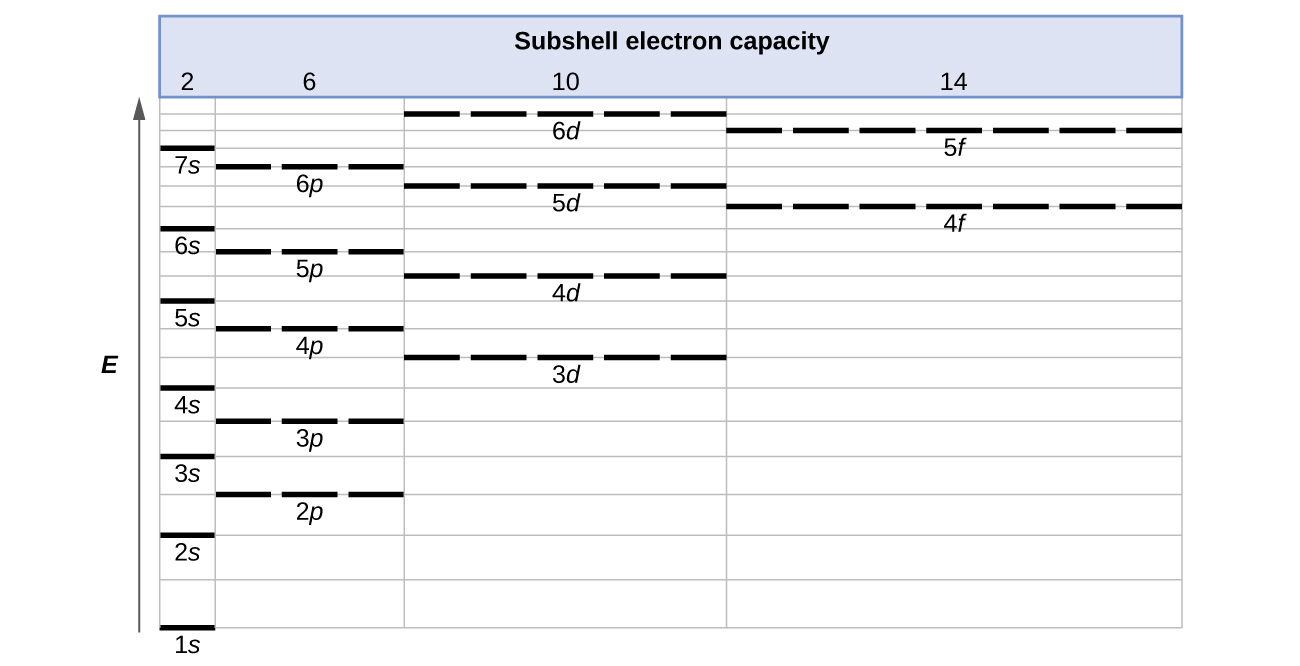6.4 Electronic structure of atoms (electron configurations)

 Page 1 / 15
By the end of this section, you will be able to:
• Derive the predicted ground-state electron configurations of atoms
• Identify and explain exceptions to predicted electron configurations for atoms and ions
• Relate electron configurations to element classifications in the periodic table

Having introduced the basics of atomic structure and quantum mechanics, we can use our understanding of quantum numbers to determine how atomic orbitals relate to one another. This allows us to determine which orbitals are occupied by electrons in each atom. The specific arrangement of electrons in orbitals of an atom determines many of the chemical properties of that atom.

Orbital energies and atomic structure

The energy of atomic orbitals increases as the principal quantum number, n , increases. In any atom with two or more electrons, the repulsion between the electrons makes energies of subshells with different values of l differ so that the energy of the orbitals increases within a shell in the order s < p < d < f. [link] depicts how these two trends in increasing energy relate. The 1 s orbital at the bottom of the diagram is the orbital with electrons of lowest energy. The energy increases as we move up to the 2 s and then 2 p , 3 s , and 3 p orbitals, showing that the increasing n value has more influence on energy than the increasing l value for small atoms. However, this pattern does not hold for larger atoms. The 3 d orbital is higher in energy than the 4 s orbital. Such overlaps continue to occur frequently as we move up the chart.Generalized energy-level diagram for atomic orbitals in an atom with two or more electrons (not to scale).

Electrons in successive atoms on the periodic table tend to fill low-energy orbitals first. Thus, many students find it confusing that, for example, the 5 p orbitals fill immediately after the 4 d , and immediately before the 6 s . The filling order is based on observed experimental results, and has been confirmed by theoretical calculations. As the principal quantum number, n , increases, the size of the orbital increases and the electrons spend more time farther from the nucleus. Thus, the attraction to the nucleus is weaker and the energy associated with the orbital is higher (less stabilized). But this is not the only effect we have to take into account. Within each shell, as the value of l increases, the electrons are less penetrating (meaning there is less electron density found close to the nucleus), in the order s > p > d > f . Electrons that are closer to the nucleus slightly repel electrons that are farther out, offsetting the more dominant electron–nucleus attractions slightly (recall that all electrons have −1 charges, but nuclei have + Z charges). This phenomenon is called shielding and will be discussed in more detail in the next section. Electrons in orbitals that experience more shielding are less stabilized and thus higher in energy. For small orbitals (1 s through 3 p ), the increase in energy due to n is more significant than the increase due to l ; however, for larger orbitals the two trends are comparable and cannot be simply predicted. We will discuss methods for remembering the observed order.

What is stoichometry
what is atom
An indivisible part of an element
ngwuebo
the smallest particle of an element which is indivisible is called an atom
Aloaye
An atom is the smallest indivisible particle of an element that can take part in chemical reaction
Alieu
is carbonates soluble
what is the difference between light and electricity
What is atom? atom can be defined as the smallest particles
what is the difference between Anode and nodes?
What's the net equations for the three steps of dissociation of phosphoric acid?
what is chemistry
the study of matter
Reginald
what did the first law of thermodynamics say
energy can neither be created or distroyed it can only be transferred or converted from one form to another
Graham's law of Diffusion
what is melting vaporization
melting and boiling point explain in term of molecular motion and Brownian movement
Anieke
Scientific notation for 150.9433962
what is aromaticity
aromaticity is a conjugated pi system specific to organic rings like benzene, which have an odd number of electron pairs within the system that allows for exceptional molecular stability
Pookieman
what is caustic soda
sodium hydroxide (NaOH)
Kamaluddeen
what is distilled water
Rihanat
is simply means a condensed water vapour
Kamaluddeen
a hydrocarbon contains 7.7 percent by mass of hydrogen and 92.3 percent by mass of carbon
how many types of covalent r there
how many covalent bond r there
JArim
they are three 3
TYPES OF COVALENT BOND-POLAR BOND-NON POLAR BOND-DOUBLE BOND-TRIPPLE BOND. There are three types of covalent bond depending upon the number of shared electron pairs. A covalent bond formed by the mutual sharing of one electron pair between two atoms is called a "Single Covalent bond.
UsmanByBy Edward Biton